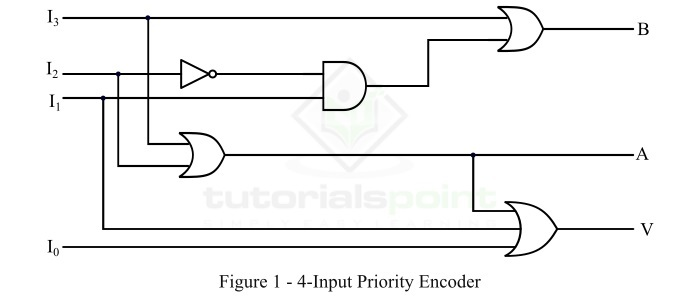# Priority Encoder in Digital Electronics

In digital electronics, an encoder is a combinational logic circuit which accepts inputs as decimal digits and alphabetic characters, and produces the outputs as the coded representation of the inputs. In other words, an electronic combinational circuit that converts numbers and symbols into their corresponding coded format is called an encoder. The operation performed by the encoder is called encoding which is a process of converting familiar numbers and characters into their equivalent codes.

An encoder has 2n input lines and n-output lines. At a time, only one of the 2n input lines is activated. The coded output of the encoder depends upon the activated input line. There are several types of encoders available such as "octal to binary encoder", "decimal to BCD encoder", "keyboard encoders", etc.

## What is a Priority Encoder?

In case of an ordinary encoder, one and only one decimal input can be activated at any given time. But in the case of some practical digital systems, two or more decimal inputs can unintentionally become active at the same time that might cause a confusion. For example, on a keyboard, a user presses key 4 before releasing another key 2. In such a situation, the output will be corresponding to (6)10, instead of being (4)10 or (2)10. This kind of problems can be solved with the help of priority encoder.

In digital electronics, a combinational logic circuit which produces outputs in response to only one input among all those that may be activated at the same time is called a priority encoder. For this, it uses a priority system, and hence it is named so.

One most popular priority system used is based on the relative magnitudes of the inputs. According to the priority system, the decimal input having largest magnitude among all the simultaneous inputs is encoded. Hence, as per this priority encoding system, the priority encoder would encode 4 if both 4 and 2 are active at the same time.

In some practical systems, priority encoders have several inputs which are routinely active at the same time. In such cases, the primary function of the encoder is to select the input with the highest priority. This function of the priority encoder is known as arbitration. For example, in a computer system, multiple input devices are connected, and several of them may try to supply data to the system at the same time. In this case, the priority encoder is responsible for enabling that input device which has the highest priority among all the input devices.

## Types of Priority Encoders

Several types of priority encoders are there. Some most important types of priority encoders are listed and explained below.

• 4 Input Priority Encoder

• Decimal to BCD Priority Encoder

• Octal to Binary Priority Encoder

Let us discuss each type of priority encoder in detail.

## 4-Input Priority Encoder

The logic circuit of the 4-input priority encoder is shown in Figure-1.It has three outputs designated by A, B, and V. Where, A and B are the ordinary outputs and V is the output that acts as a valid bit indicator. This third output V is set to 1 when one or more inputs are equal to 1. In the case, when all the inputs to the encoder are equal to 0, there is no any valid input, and thus the output V is set to 0. The other two outputs, i.e. A and B of the encoder are not determined when V is equal to 0. Therefore, when, V is equal to 0, the outputs A and B are specified as "don’t care conditions".

The truth table of the 4-input priority encoder is shown below.

Inputs (× = Don’t care) Outputs
I0 I1 I2 I3ABV
0000××0
1000001
×100011
××10101
×××1111

From this truth table, it can be observed that the higher the subscript number of the input, the higher the priority of the input. Thus, the input I3 has the highest priority. Therefore, regardless of the values of other inputs, when the input I3 is equal to 1, the output for AB is 11, i.e. 3. The input I2 has the next lower priority, and then I1, and finally I0 has the lowest priority.

We can write the Boolean expression for outputs A, B, and V from the above table as follows,

$$A=I_{3}+\bar{I_{3}}I_{2}=I_{3}+I_{2}$$

$$B=I_{3}+\bar{I_{3}}\bar{I_{2}}I_{1}=I_{3}+\bar{I_{2}}I_{1}$$

And,

$$V=I_{3}+I_{2}+I_{1}+I_{0}$$

Hence, the condition for the output V is an OR operation of all the input variables.

## Decimal to BCD Priority Encoder

This type of priority encoder performs the function of encoding the decimal digits into 4-bit BCD (Binary Coded Decimal) outputs. As it is a decimal to BCD priority encoder, therefore, it produces a BCD corresponding to the decimal digit of highest priority among all the inputs and ignores all others.

The truth table of the decimal to BCD priority encoder is given below.

Decimal Inputs (× = Don’t care) BCD Outputs
I1 I2 I3 I4 I5 I6 I7 I8 I9 A3 A2 A1 A0
1111111111111
××××××××00110
×××××××010111
××××××0111000
×××××01111001
××××011111010
×××0111111011
××01111111100
×011111111101
0111111111110

The truth table of the decimal to BCD priority encoder clearly shows that the magnitudes of the decimal inputs determine their priorities. If any decimal input is HIGH, it will be encoded if all other higher value inputs are LOW regardless of the state of all lower value inputs.

## Octal to Binary Priority Encoder

This type of priority encoder is used to perform encoding of octal code into binary code. Hence, this type priority encoder has eight inputs and three outputs that produce corresponding binary code as given in the truth table below.

Inputs Outputs
I0 I1 I2 I3 I4 I5 I6 I7 A2 A1 A0 V
00000000×××0
100000000001
×10000000011
××1000000101
×××100000111
××××10001001
×××××1001011
××××××101101
×××××××11111

This is all about the priority encoder and its major types in digital electronics.

Updated on: 22-Aug-2023

715 Views